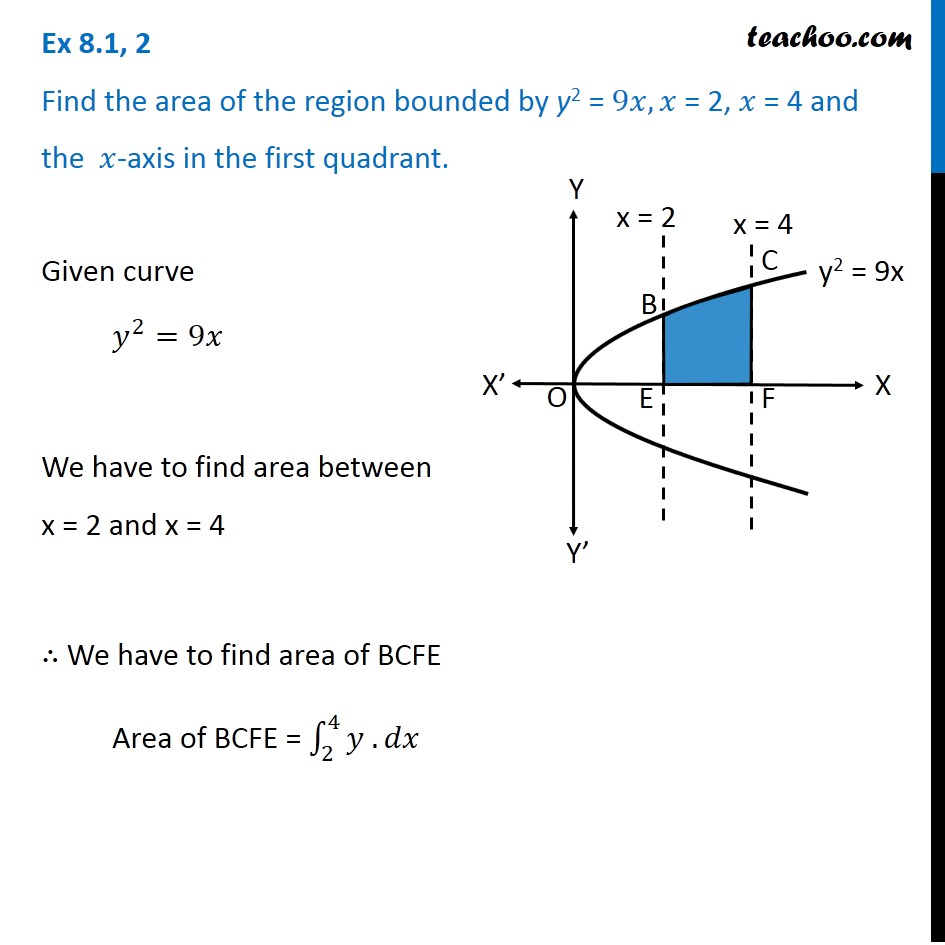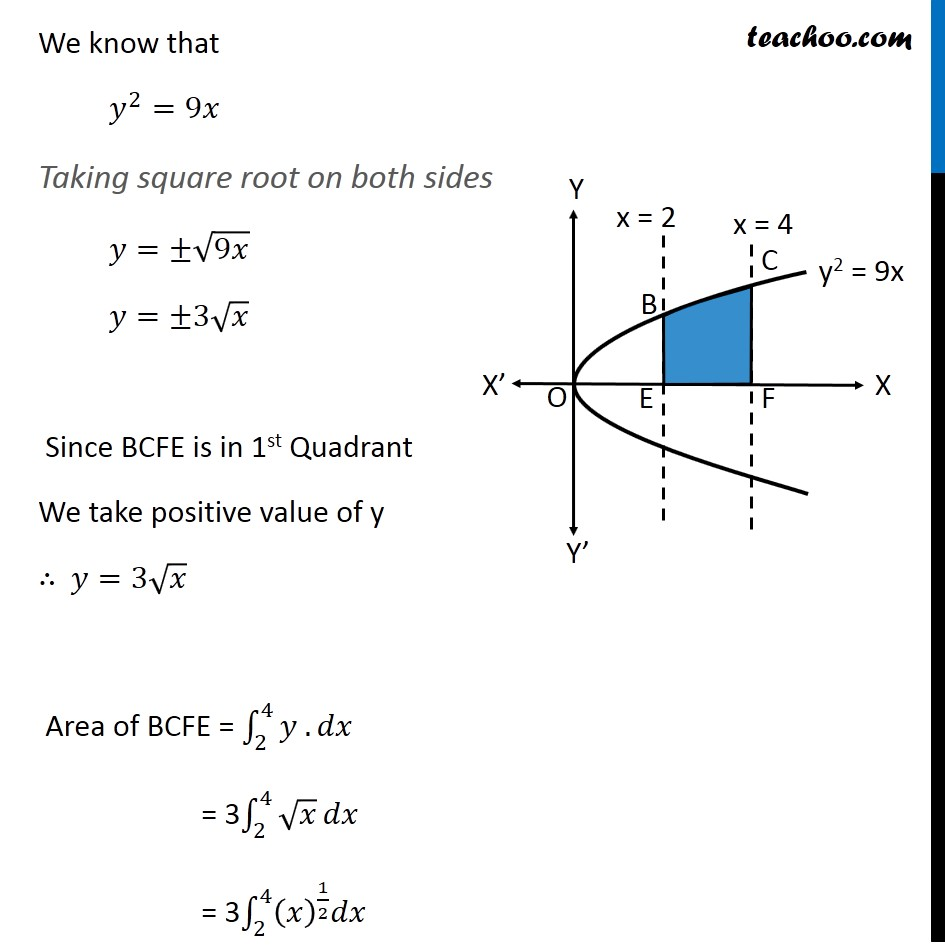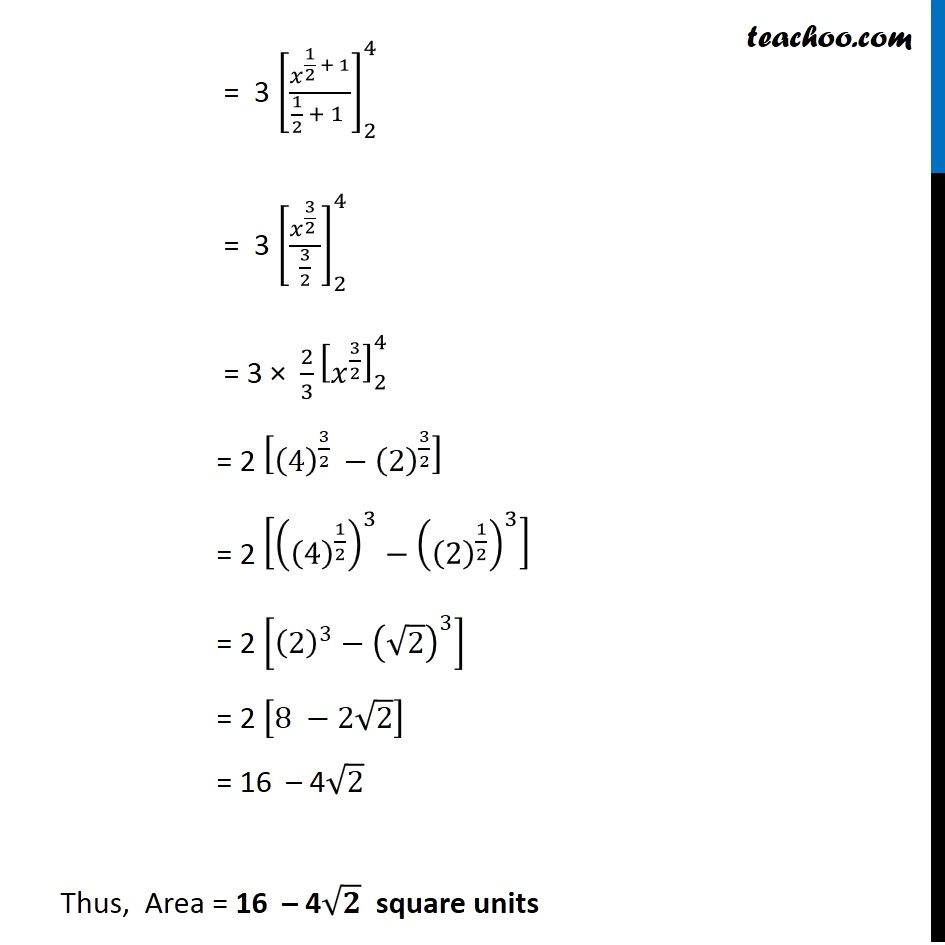Area bounded by curve and horizontal or vertical line

Chapter 8 Class 12 Application of Integrals
Concept wiseLearn in your speed, with individual attention - Teachoo Maths 1-on-1 Class

### Transcript

Question 2 Find the area of the region bounded by y2 = 9𝑥, 𝑥 = 2, 𝑥 = 4 and the 𝑥-axis in the first quadrant. Given curve 𝑦^2=9𝑥 We have to find area between x = 2 and x = 4 ∴ We have to find area of BCFE Area of BCFE = ∫_2^4▒〖𝑦 .〗 𝑑𝑥 We know that 𝑦^2=9𝑥 Taking square root on both sides 𝑦=±√9𝑥 𝑦=±3√𝑥 Since BCFE is in 1st Quadrant We take positive value of y ∴ 𝑦=3√𝑥 Area of BCFE = ∫_2^4▒〖𝑦 .〗 𝑑𝑥 = 3∫_2^4▒√𝑥 𝑑𝑥 = 3∫_2^4▒〖(𝑥)^(1/2) 𝑑𝑥〗 = 3 [𝑥^(1/2 + 1)/(1/2 + 1 )]_2^4 = 3 [𝑥^(3/2 )/(3/2)]_2^4 = 3 × 2/3 [𝑥^(3/2) ]_2^4 = 2 [(4)^(3/2 )−(2)^(3/2) ] = 2 [((4)^(1/2) )^3−((2)^(1/2) )^3 ] = 2 [(2)^3−(√2)^3 ] = 2 [8 −2√2] = 16 – 4√2 Thus, Area = 16 – 4√𝟐 square units